Trusted answers to developer questions
Trusted Answers to Developer Questions

Related Tags

canvas
javascript
communitycreator

# How to draw circles and arcs in HTML Canvas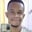Chidume Nnamdi

Grokking Modern System Design Interview for Engineers & Managers

Ace your System Design Interview and take your career to the next level. Learn to handle the design of applications like Netflix, Quora, Facebook, Uber, and many more in a 45-min interview. Learn the RESHADED framework for architecting web-scale applications by determining requirements, constraints, and assumptions before diving into a step-by-step design process.

### Overview

canvas has APIs to draw circles and arcs:

• arc(x, y, radius, startAngle, endAngle, anticlockwise): Draws an arc that is centered at (x, y) position with radius r starting at startAngle and ending at endAngle, going in the given direction indicated by anticlockwise (defaulting to clockwise).

• arcTo(x1, y1, x2, y2, radius): Draws an arc with the given control points and radius, connected to the previous point by a straight line.

Note: The radius is in radians, not degrees. $180$ degrees should be converted to radians or the APIs will take it as $180$rads. We can convert degrees to radians with this algorithm: $(radians =(Math.PI/180)*degrees)$.

In the methods above, $x, y, x1, y1$ are the center position of the circle/arc on the canvas grid. radius is the radius of the circle/arc. startAngle is the angle (in radians) where the drawing of the circle/arc will start. endAngle is the angle (in radians) where the tracing of the circle/arc would stop. antiClockWise is the direction of the circle/arc tracing. If antiClockWise is true, the circle/arc is drawn anti-clockwise; otherwise, clockwise.

Since the methods are all arcs, drawing a circle with them is a little tricky.

### Example

const degToRad = (degrees) => (Math.PI / 180) * degrees

context.stroke()


This will draw a circle.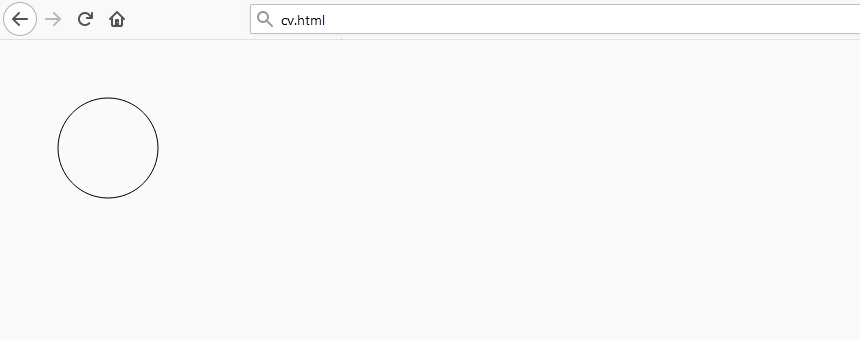### Explanation

We set the center of the circle to start at $x,y$ = $(100,100)$, with a radius of $50$. We set the starting angle at $0$ degrees (we use the degToRag function to convert degrees to radians) and the stop angle at $360$ degrees to make the complete circle. Then, the argument true makes the code trace the circle from the anticlockwise direction. The stroke() method draws out the outline of the circle.

### Examples

Let’s fill the circle through the fill method:

context.arc(100, 100, 50, degToRad(0), degToRad(360), true)
context.fill()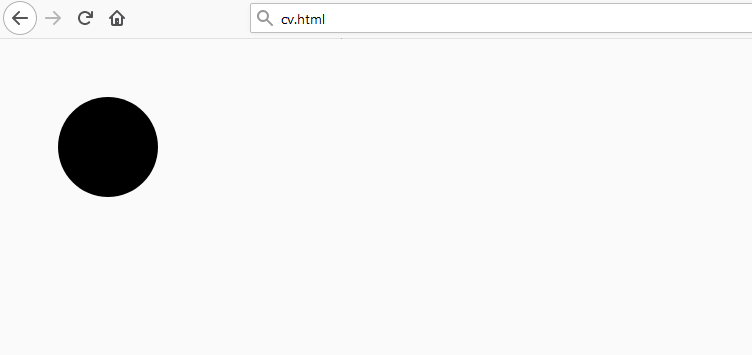We can draw an arc from $0$ to $180$:

context.arc(100, 100, 50, degToRad(0), degToRad(180), true)
context.stroke()


This will draw an arc: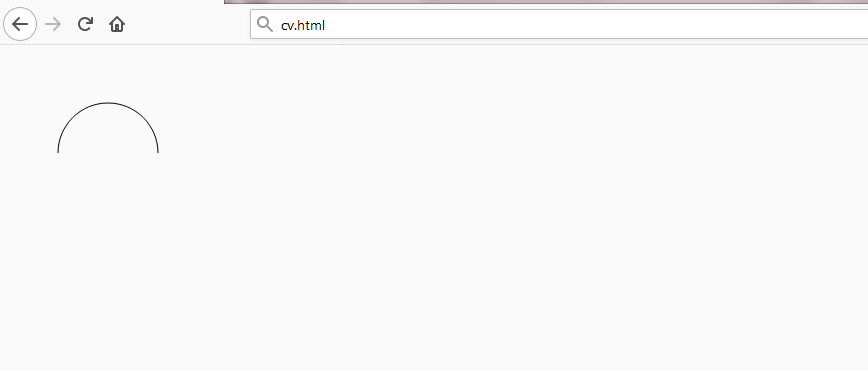This is because we start at angle $0$ and stop at $180$. If we change $180$ to $360$, the code will draw a circle.

Now, let’s make a filled arc:

context.arc(100, 100, 50, degToRad(0), degToRad(180), true)
context.fill()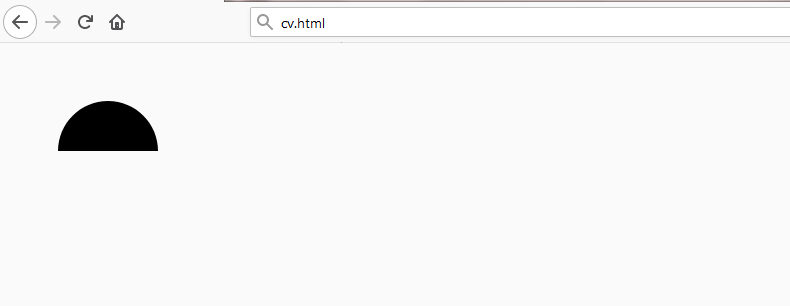.

Note: Please refer to the following shots for information related to this topic:
Drawing lines and paths
Drawing triangles
Filling paths
Drawing rectangles

RELATED TAGS

canvas
javascript
communitycreator

CONTRIBUTOR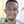Chidume Nnamdi

Grokking Modern System Design Interview for Engineers & Managers

Ace your System Design Interview and take your career to the next level. Learn to handle the design of applications like Netflix, Quora, Facebook, Uber, and many more in a 45-min interview. Learn the RESHADED framework for architecting web-scale applications by determining requirements, constraints, and assumptions before diving into a step-by-step design process.

Keep Exploring

Learn in-demand tech skills in half the time